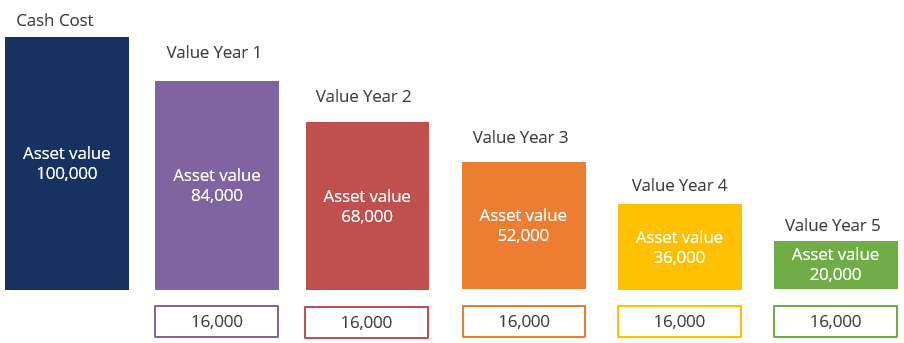## What is “Depreciation”?

Depreciation is a method of allocating the cost of a tangible asset over its useful economic life. It represents the consumption of benefits over time and matches the revenues in any period with the asset’s cost of producing those revenues. It is a non-cash item expensed through the income statement and does not represent a decline in the market value of the asset.

Businesses depreciate non-current assets for accounting purposes. For example, a business purchasing a new machine would initially record this in its balance sheet as an asset. Instead of realizing the entire cost of the asset in year one through the income statement, depreciating the asset allows the business to spread out that cost and generate revenue from it.

## Key Learning Points

• Depreciation is a non-cash expense and represents the consumption of benefits of a tangible asset over time
• Depreciation decreases the net asset value reported in the balance sheet but does not represent a decline in the market value of the asset
• Straight-line depreciation is the most common method used by accountants and charges the same expense each year of the asset’s life
• Companies report PP&E at their net book value which is the cost of the asset less accumulated depreciation, accumulated amortization, accumulated depletion or accumulated impairment

## How is Depreciation Calculated

There are many methods of calculating depreciation but there are two main systems, straight line and reducing balance. The straight-line method is the most widely used and is quick and easy to calculate.

Depreciation Expense = (Cost – Salvage Value) / Useful Life of Asset

Here we take the initial cost of the asset and reduce this by its salvage value (the estimated value of the asset at the end of its life expectancy). Dividing this by the number of years the asset is expected to be used gives the depreciation expense for each year. With this method, the depreciation expense is the same for each accounting period.

There are a number of different methods for allocating the depreciation expense, including reducing balance and double declining. These follow the same principle as straight line where the asset is expensed across its life but with varying depreciation expenses. No matter which method is chosen, the total amount of depreciation charged should be the same: The difference between the cost of the asset and its residual or scrap value. The difference is in the timing. Some methods charge more depreciation towards the start (reducing balance), whereas others gradually charge the same every year (straight line).

## Depreciation in the Financial Statements

A company purchases a new long-term asset which increases the asset account on the balance sheet. This causes a decrease in cash. Neither entries affect the income statement but instead, the asset cost is expensed through regular depreciation charges across the asset’s useful life.

When the business believes that the carrying amount of PP&E is overstated, it should impair or write down the book value of the asset to fair value. This may be due to an unexpected change in market conditions or other circumstances. Fair value is the anticipated recoverable amount. This process is called an impairment and is fundamentally like unscheduled depreciation. The carrying amount of the asset is reduced and the income statement is expensed. These charges can be significant and are impossible to forecast. They are normally considered non-recurring for the purposes of normalizing profits.

## Example of Depreciation

A company purchases an asset for 100,000. It could expense the entire cost of the asset in year 1 or write down the asset’s value across its useful life. For this example, let’s assume the asset has a life expectancy of 5 years. The company expects to scrap the asset for 20,000 at the end of its life. What is the effect on the company’s financial statements using the straight-line method?The company records a cash cost of 100,000 on its balance sheet. The next step is to calculate the depreciation expense incurred each year, which is the cost of the asset less its salvage value, divided by the useful life of the asset. The calculation becomes (100,000 – 20,000) / 5, which is a 16,000 depreciation charge per year.

The 16,000 charge is expensed through the income statement reducing the assets carrying value amount. The asset value recorded in the balance sheet will be the gross value of the asset minus accumulated depreciation.